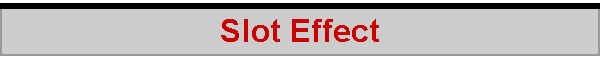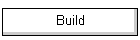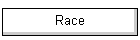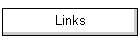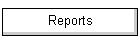On the beat, the jib is sheeted at a larger angle to the apparent wind than is the main. On the IOM, the jib sheeting angle would be somewhere around 12 or 15 degrees, while the main sheeting angle might be 3 or 5 degrees. The difference in sheeting angles forms the "slot", that gap between jib and main whose quantitative performance seems to be poorly understood.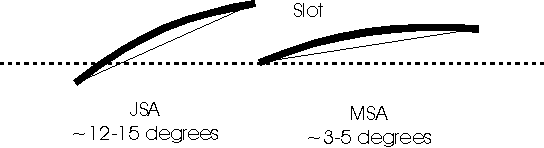Qualitatively, the main is sheeted more closely than the jib because the effect of the jib is to place the main into a headed wind. While a jib sheeting angle of 12 or 15 degrees gives the jib an angle of attack of 18 to 15 degrees to an apparent wind angled at, say, 30 degrees, a sheeting angle of 3 or 5 degrees is needed at the main because the apparent wind has been deflected by the jib by an angle of, say, 10 degrees, and now approaches the main at an apparent angle of 20 degrees. The main needs to be sheeted at 3 or 5 degrees in order to maintain its own angle of attack of about 17 or 15 degrees to the oncoming wind. (The jib has a slightly higher angle of attack than the main because it generally has slightly less draft, and so has an entry angle that needs the slightly higher angle of attack. Have a look at the spreadsheet for entry and exit angles to play with these parameters.) Two questions now arise. First, exactly how much does the jib deflect the wind? Second, as the sheet is eased for the reach and the wind moves abeam, exactly how much of the slot effect remains? My answer to these questions is highly speculative, since I've not seen any quantitative research on the topic or any treatment in the technical books. One approach is to assume that the wind is deflected by the jib in proportion to the exit angle on the jib leech. (Use the spreadsheet for entry and exit angles to play with exit angles.) The "full" exit angle would deflect the wind more than is observed in practice, though, so a "fudge factor" is needed to reduce the theoretical deflection so that it better matches observation. The slot spreadsheet model (about 24kb) calculates the "slot effect". The "fudge factor" is called a "jib area discount", and a value of 50% seems to work. I've shrugged and told myself that the main is deflecting the air up towards the jib about as much as the jib is deflecting the air down towards the main, so a jib effectiveness of 50% seems about right...! The "jib area discount" fudge factor will also be used to determine the slot effect higher up the mast, and not just at boom level. In this case, of course, the slot effectively vanishes at the hounds, and the "discount" is 0% -- ie no slot effect because there is no "jib area" to deflect the wind.Then there is a factor which gives a maximum wind deflection when the jib is sheeted in to the same angle as the main, and a minimum deflection when the jib is fully sheeted out as on a very broad reach. I've decided that this factor is an "inverse square law" factor, based on the momentum of the air that is passing through the slot. The sine of the jib sheeting angle is a measure of the size of the slot, so the spreadsheet takes the square root of this sine and subtracts it from unity. This factor is called the "slot gap index". Finally, the spreadsheet considers a relatively minor factor to do with wind velocity. In light airs, the slot is probably slightly more effective, and in heavy airs it is also probably more effective. The spreadsheet allows a setting to be made of the wind speed at which the slot is thought to be least effective (3m/s in the model), and a percentage factor which increases the slot effectiveness as the wind either rises or falls around this value (10% in the model). Given these assumptions, the spreadsheet model shows that the slot effect decays quite rapidly. Close hauled (apparent wind at about 30 degrees), the jib deflects the wind by 7 or 8 degrees. On a close reach with an apparent wind angle of about 60 degrees, the wind deflection is about 2 or 3 degrees. On a broad reach with an apparent wind angle of about 90 degrees, wind deflection is effectively zero. (Another approach is shown on the twist page, where a rough but perhaps better estimate is made of the downwash behind the jib.) 2005-12-18